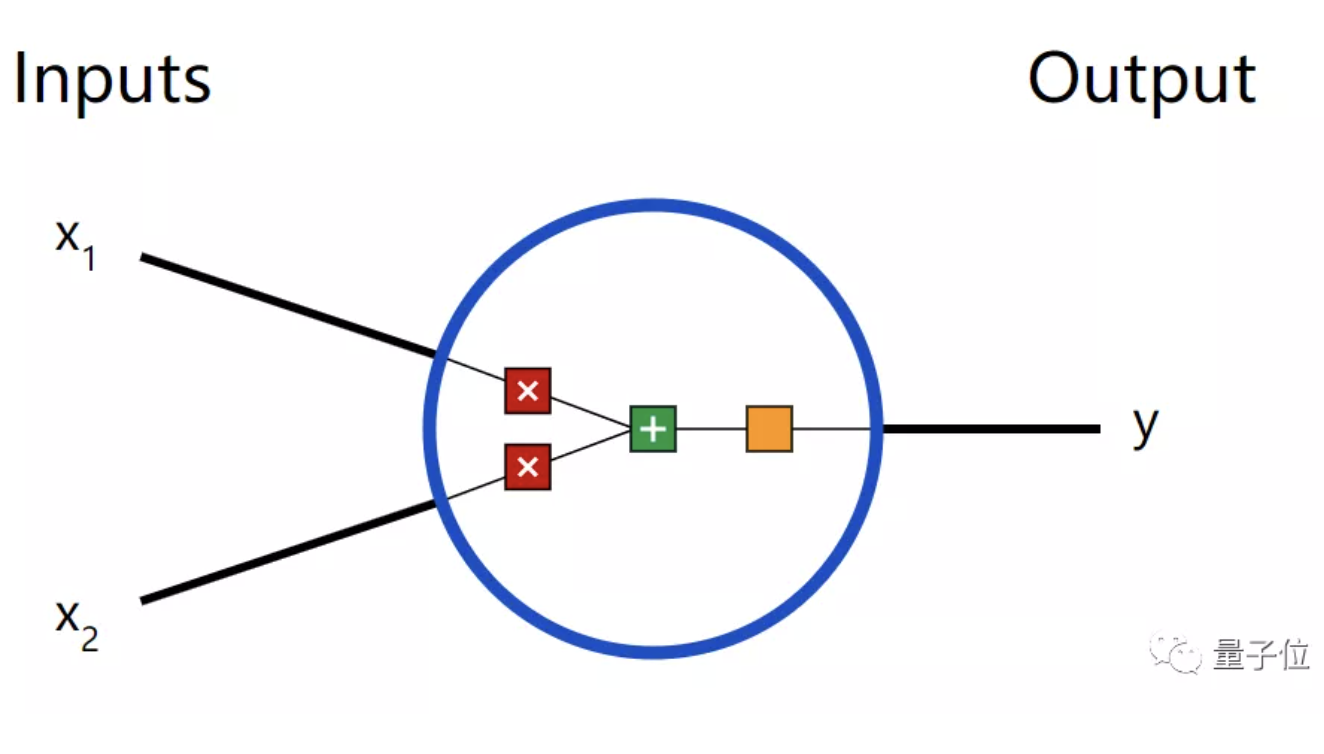# 神经网络原来这么简单，机器学习入门贴送给你 | 干货## 神经网络概论

“神经网络”一词很流行，人们通常认为它很难，但其实要简单得多。

### 神经元——神经网络的基本单元1、每个输入乘以相应的权重；2、将所有加权输入加在一起，在加上一个偏差b；3、导入一个激活函数，得到输出y。input：x=[2,3]

output：y=0.999``````import numpy as np

def sigmoid(x):
# Our activation function: f(x) = 1 / (1 + e^(-x))
return 1 / (1 + np.exp(-x))

class Neuron:
def __init__(self, weights, bias):
self.weights = weights
self.bias = bias

def feedforward(self, inputs):
# Weight inputs, add bias, then use the activation function
total = np.dot(self.weights, inputs) + self.bias
return sigmoid(total)

weights = np.array([0, 1]) # w1 = 0, w2 = 1
bias = 4                   # b = 4
n = Neuron(weights, bias)

x = np.array([2, 3])       # x1 = 2, x2 = 3
print(n.feedforward(x))    # 0.9990889488055994``````

### 构建神经网络``````import numpy as np

# ... code from previous section here

class OurNeuralNetwork:
'''
A neural network with:
- 2 inputs
- a hidden layer with 2 neurons (h1, h2)
- an output layer with 1 neuron (o1)
Each neuron has the same weights and bias:
- w = [0, 1]
- b = 0
'''
def __init__(self):
weights = np.array([0, 1])
bias = 0

# The Neuron class here is from the previous section
self.h1 = Neuron(weights, bias)
self.h2 = Neuron(weights, bias)
self.o1 = Neuron(weights, bias)

def feedforward(self, x):
out_h1 = self.h1.feedforward(x)
out_h2 = self.h2.feedforward(x)

# The inputs for o1 are the outputs from h1 and h2
out_o1 = self.o1.feedforward(np.array([out_h1, out_h2]))

return out_o1

network = OurNeuralNetwork()
x = np.array([2, 3])
print(network.feedforward(x)) # 0.7216325609518421``````

### 训练神经网路——计算损失函数Male设置为0，Female设置为1，还对其余数据进行了简化。Python示例：

``````import numpy as np

def mse_loss(y_true, y_pred):
# y_true and y_pred are numpy arrays of the same length.
return ((y_true - y_pred) ** 2).mean()

y_true = np.array([1, 0, 0, 1])
y_pred = np.array([0, 0, 0, 0])

print(mse_loss(y_true, y_pred)) # 0.5``````

### 训练神经网络——最小化损失1、数据集中选择一个样本，就如Alice。

2、利用反向传播计算所有变量的偏导数。

3、使用随机梯度下降来训练神经网络，更新变量。

4、返回步骤1。

## 传送门

https://victorzhou.com/tag/machine-learning/